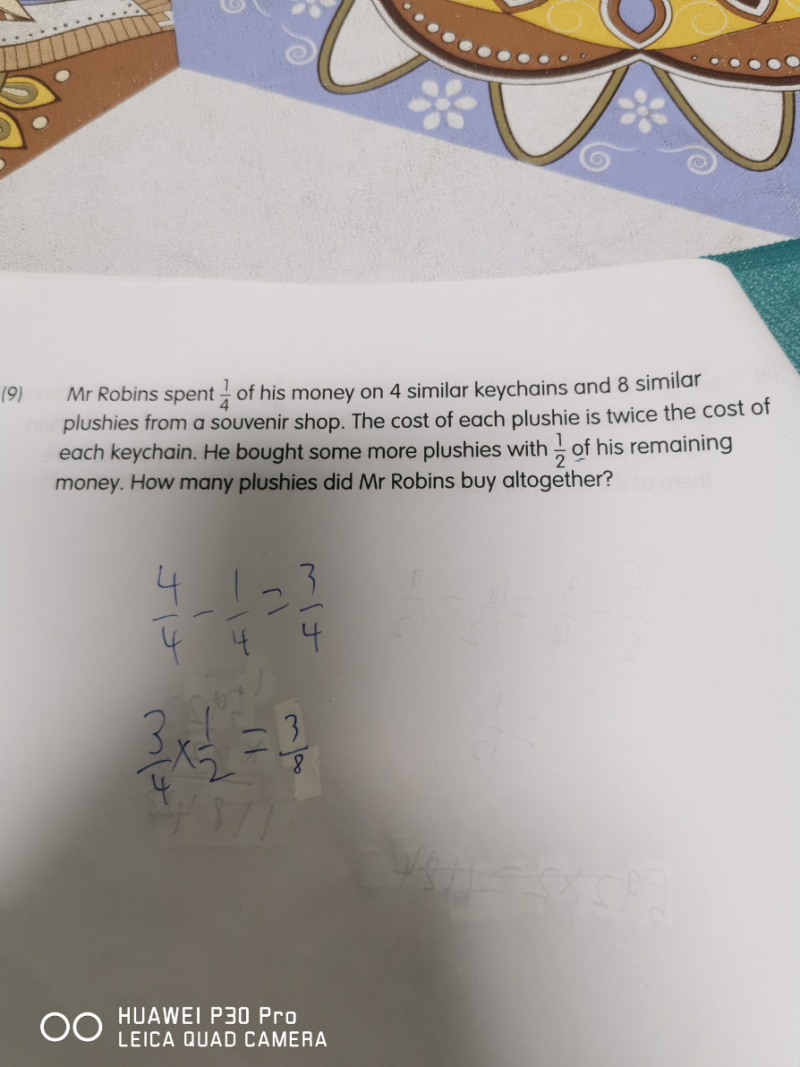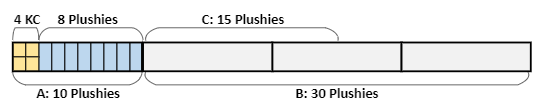# QuestionSource: Keming Primary

Unit cost

Plushie        [u][u]

Keychain      [u]

4u + 8(2u) = 20u          Remainder money= (3/4)        Buy more plushies with ( 1/2) (3/4) =  (3/8)

1/4 money  spent  –>  20u

3/8 money spent  –> (20u x 4)  x  (3/8) = 30u

1 plushie  -> 2u   =>  30u/2u = 15 plushies

15+8 = 23

Hence Mr Robins bought 23 plushies altogether.

0 Replies 1 Like
`Here is a block diagram solution:````Explanation
A: 1 plushie (blue) can buy to 2 keychain (yellow).
So we see that 1/4 of his money can buy 10 plushies.
B: The remaining of his money can buy 3 x 10 = 30 plushies.
C: So half of the remaining money can buy 30/2 = 15 plushies.

Answer: He bought 8 + 15 = 23 plushies.```
0 Replies 2 Likes

If each keychain costs L, each plushie costs 2L

Then ¼ of money can buy 4L+8*2L= 20L

Half of the remaining money = ½*(1-1/4)=3/8 which is 3/2*1/4 or you can understand it as 1.5 times what ¼ of money can buy.

So Half of the remaining money  can buy 1.5*20L=30L and can buy 15 more plushies.

So in total Mr Robin bought 15+8=23 plushies altogether.

0 Replies 1 Like